## How to build a passive crossover ?

Follow a passive crossover schematic, and build a basic electrical circuit. It’s that easy. Before we get into that, you first have to know what are your needs, and which type of crossover is more suited for your application. If you haven’t already, please read this article to get a basic idea on how crossover networks work. Next, let’s recap the categories of the crossovers :

You got the order of the crossover which dictates the slope :

• 1st order – 6 dB / octave slope.
• 2nd order – 12 dB / octave slope.
• 3rd order – 18 dB / octave slope.
• 4th order – 24 dB / octave slope.
• etc.

And then you got the type :

• Linkwitz-Riley crossover – which matches the attenuation slopes, so they combine for a flat response at the crossover point.
• Butterworth crossover – which creates a small peak in response at the crossover point.
• Bessel crossover – is somewhere between the first two.

Depending on how much protection you need for your drivers, you will pick your crossover order to determine the steepness of the attenuation slope. If, for example, you need to set a crossover point for a tweeter, then depending on it’s usable frequency range, you will select a crossover point and the slope accordingly. If you select a shallow slope (1st order), the tweeter will reach the lower octaves as well, and depending on it’s playable frequency range, it could damage the tweeter. On the other hand, bear in mind that going for a higher order crossover, will make the design more complex. In fact, the order of the x-over will determine the amount of capacitors / inductors the design will have. In general, the more components the crossover has, the worse it gets for the audio signal. Go for a lower order crossover whenever possible.

### Passive crossover schematic for multi-way networks

Soon, we will get into detail about the types and the passive crossover schematic of each one in particular. When we will discuss the crossover types, we will be talking about the 2-way setup as it is the most common. However, 3-way setups are not that uncommon. 4 or more way systems are usually pretty scarce. Let’s talk about the 3 way crossover, so you can understand how multi-way works, and then we will go into details about the crossover types, focusing on 2 way systems.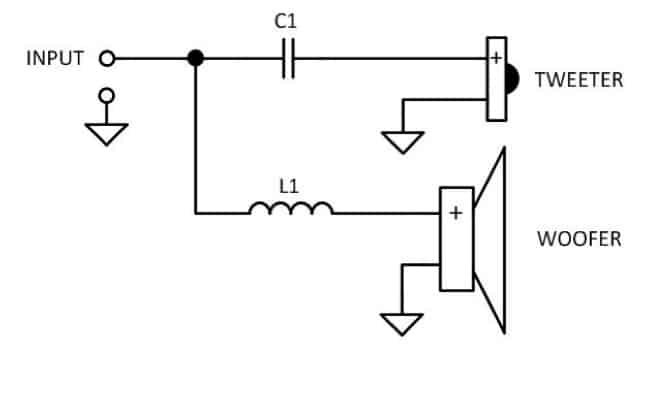This is the simplest crossover design out there, for a 2 way system. When you put a capacitor in series with a speaker, the lower frequencies are filtered. When you put an inductor in series with a speaker, the higher frequencies are filtered. Depending and the values of the capacitors and inductors (measured in farads and henries), various crossover points are chosen. Now to make this into a 3 way setup, the high frequencies are split once more into high and low bands. The “low” for the second split will correspond for the midrange driver.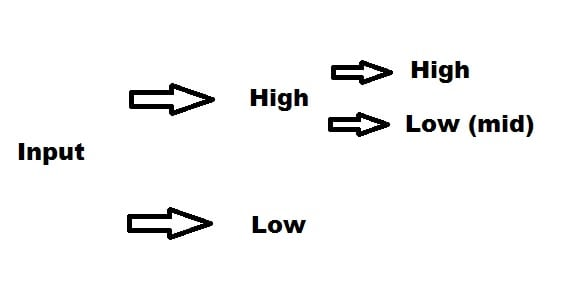Now you can split the high frequencies, once again, into high and low to get a 4 way crossover, and so on, depending on how many drivers your system has.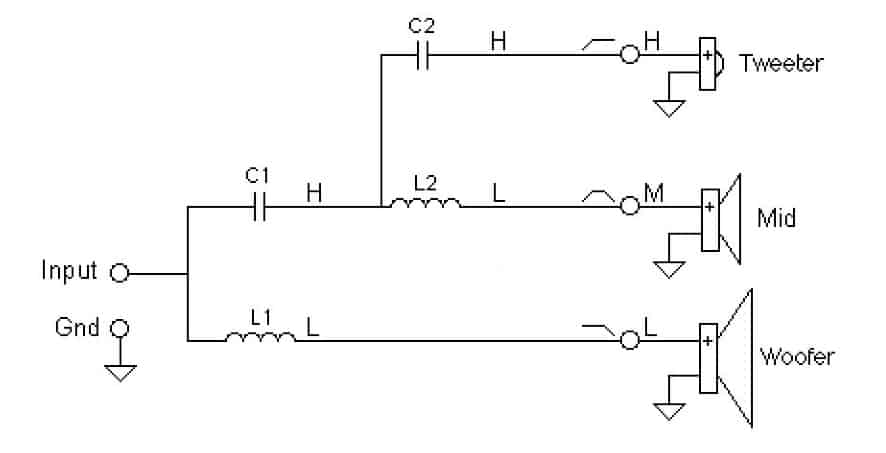To get a more realistic idea, you can see above, how the most simple 3-way crossover will look like.

### 1st order Butteworth

This is the exact same crossover as above. It is the simplest crossover design and very popular in hi-fi audio systems, with low power capabilities. Furthermore, the frequency slopes of the drivers used in the system will sum nicely, to give an accurate frequency response and delay response. Can be used with high chance of success in 3-way or multi-way systems. However, if the audio system has a high power demand, this may not be an optimal choice. Because of the shallow slope of 6 dB, the tweeter might be damaged in stressful situations, because the x-over does not provide appropriate protection. Up next, is the passive crossover schematic for a basic 1st order x-over :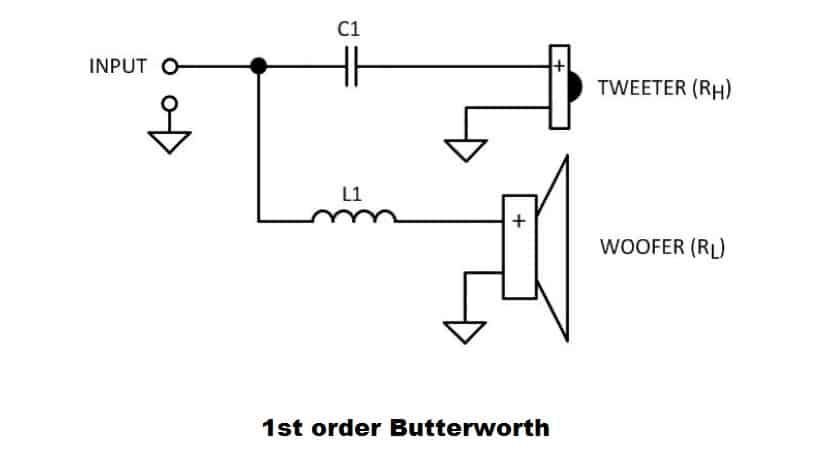To find out the values of the capacitor and inductor, you will need to know the impedance of the speakers and the desired frequency where you want to set the crossover point.

Explanation of terms :

• C1 – Capacitor 1, measured in Farads.
• L1 – Inductor 1, measured in Henries.
• RH – The rated impedance of the high frequency speaker (tweeter), measured in ohms.
• RL – The rated impedance of the low frequency speaker (woofer), measured in ohms.
• fC – crossover frequency point.

To calculate the values of the capacitor and inductor use these formulas :

• C1 = 1 / (2π * fC * RH) .
• L1 = RL / (2π * fC) .

### 2nd order Butterworth

The 2nd order crossover design has many types, because it has an adjustable Q factor. The design is the same for whatever type it is, only the values of the components will vary, which will determine the exact type of the crossover. When we are talking about the 2nd order Butterworth design, few problems appear. For the Butterworth alignment, the individual filters are 3 dB down at the crossover frequency. This means when you sum them up, you will get a dip of 3 dB at the crossover point. To counter this, you can reverse the polarity and the graph will shift upward, and you will get a peak of 3 dB at the crossover point. Generally this type of crossover is not recommended. Here is the passive crossover schematic for the 2nd order design :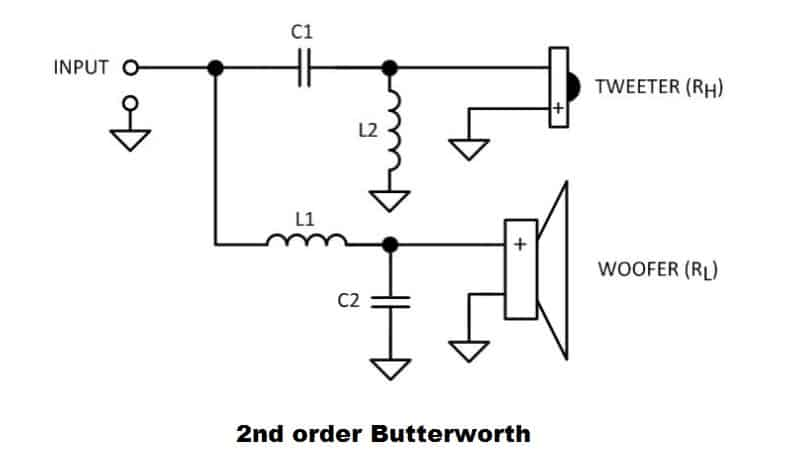As you can see, because we got higher in the order ranking, the amount of components needed has increased by 2. To calculate the values of the components use the following formulas :

• C1 = √2 / (4π * fC * RH) .
• C2 = √2 / (4π * fC * RL) .
• L1 = (√2 * RL) / (2π * fC) .
• L2 = (√2 * RH) / (2π * fC) .

Linkwitz-Riley crossover can be found in all even orders. This means there are 2nd order, 4th order, 6th order and so on, variants. What makes LR crossovers so special, is that the two frequency curves will sum up to a flat response. If we are comparing this design to Butterworth, the following differences appear : The individual Butterworth filters are 3 dB down at crossover point and LR is 6 dB down.

### How to design loudspeakers - video courses

Butterworth has 90 degree phase difference which means the 2 curves will add up for 3 dB boost. However, Linkwitz-Riley has no phase difference and the 2 curves add up for a 6 dB boost. Linkwitz-Riley is also called Butterworth squared, because it is equal to two Butterworth of lower order in series. For example two 1st order Butterworth in series is equal to 2nd order Linkwitz-Riley, or two 2nd order Butterworth in series is equal to a 4th order Linkwitz-Riley, and so on.

Regarding the 2nd order Linkwitz-Riley, the passive crossover schematic is the same as 2nd order Butterworth, but the values of the components are different. To calculate the values of the components, use these formulas :

• C1 = 1 / (4π * fC * RH) .
• C2 = 1 / (4π * fC * RL) .
• L1 = RL / (π * fC) .
• L2 = RH / (π * fC) .

### 3rd order Butterworth

This is a good solution for a 2-way system with a 18 dB / octave roll-off slope. When the high and low curves add up, they will sum for a flat amplitude, regardless of the relative polarity of the drivers. However, because the 3rd order Butterworth cannot deliver a flat delay response, they will produce frequency response problems for a multi-way system. If you want to use this filter for a 3 way setup, make sure you space out the two crossover points as much as possible.

For this design, it is necessary to inverse the polarity of the tweeter, to get the best summed phase response. Here is the passive crossover schematic for this design (note the tweeter polarity is reversed) :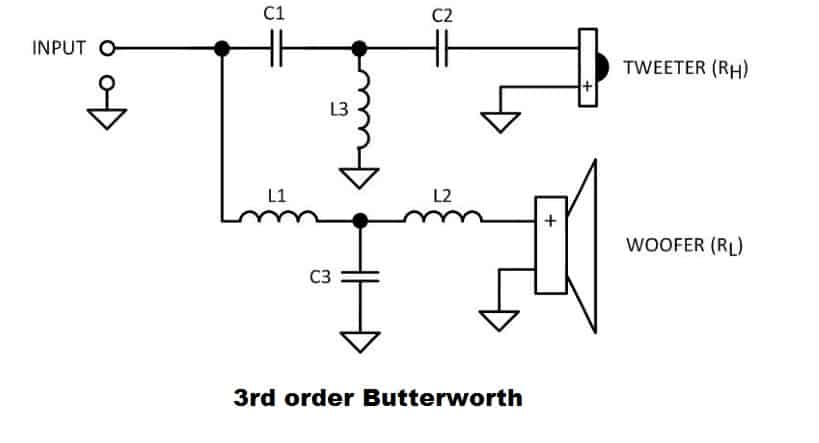To calculate the values of the components, use these formulas :

• C1 = 1 / (3π * fC * RH) .
• C2 = 1 / (π * fC * RH) .
• C3 = 2 / (3π * fC * RL) .
• L1 = 3RL / (4π * fC) .
• L2 = RL / (4π * fC) .
• L3 = 3RH / (8π * fC) .

The 4th order Linkwitz-Riley crossover is a very complex design, as it incorporates a large amount of components, and it is on the expensive side as well. It gives a flat summed frequency response, and because of the steep 24 dB / octave slope, it gives an excellent protection for the tweeters. This type does not exhibit a flat phase response, so in a multi-way setup it can cause some problems. This type of crossover is recommended over the 3rd and 4th order Butterworth due to its superior summed frequency response and desirable lobbing characteristics.

Here is the passive crossover schematic for the LR4 and how to calculate the values of the components :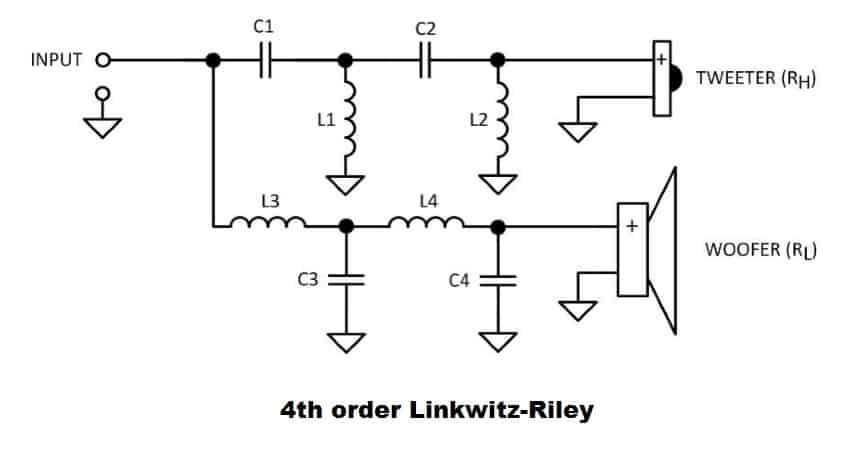• C1 = 3√2 / (16π * fC * RH) .
• C2 = 3√2 / (8π * fC * RH) .
• C3 = 9√2 / (16π * fC * RL) .
• C4 = √2 / (8π * fC * RL) .
• L1 = (2√2 * RH) / (9π * fC) .
• L2 = (√2 * RH) / (π * fC) .
• L3 = (2√2 * RL) / (3π * fC) .
• L4 = (√2 * RL) / (3π * fC) .

### Conclusion

The only crossover types which provide flat sum for both frequency response and phase response are the 1st order Butterworth or other 1st order variants. They are also the least complex and the cheapest. On the other hand, they don’t provide adequate protection for the tweeters in a high power audio system. The 2nd and 4th order Linkwitz-Riley are great for almost all 2-way systems. For a 3-way setup, at least one of the crossovers should be a constant voltage type, in order to achieve the most accurate summed frequency response. In a passive crossover network, it is not uncommon to stumble upon other circuits, besides the filter parts, like : impedance compensation networks, attenuators to match the levels of the drivers, tweeter protection circuit etc.

#### References

1. Introduction to Loudspeaker Design: Second Edition by John L. Murphy (True Audio, 2014). (Amazon affiliate link)